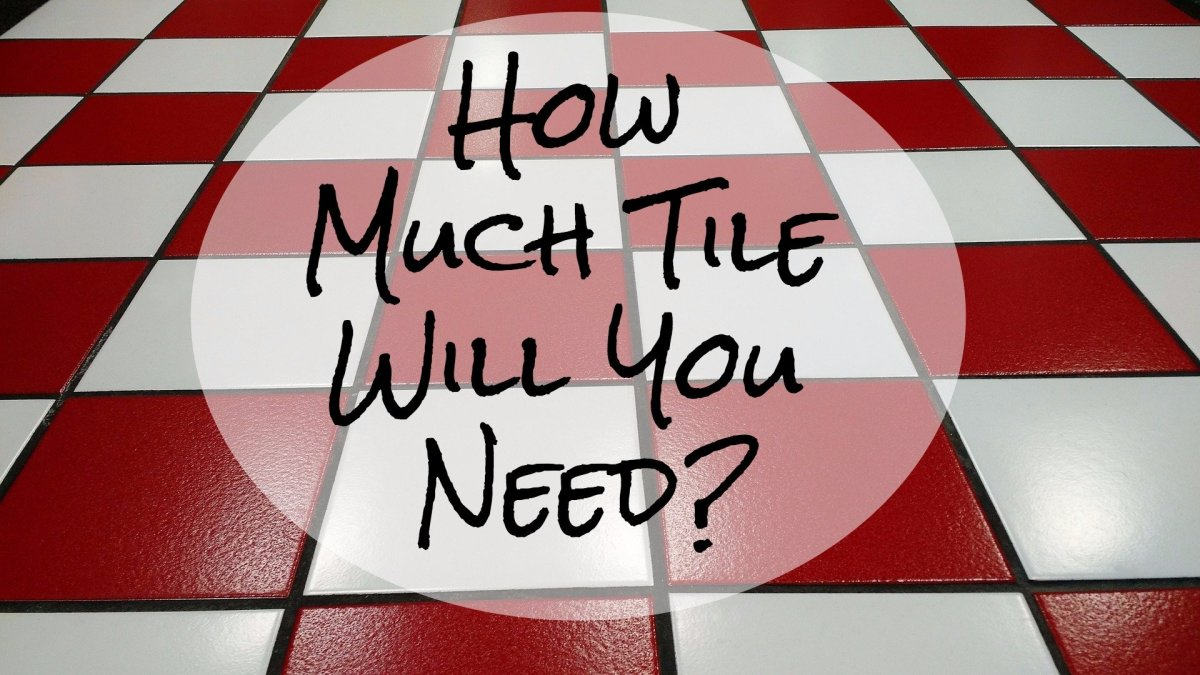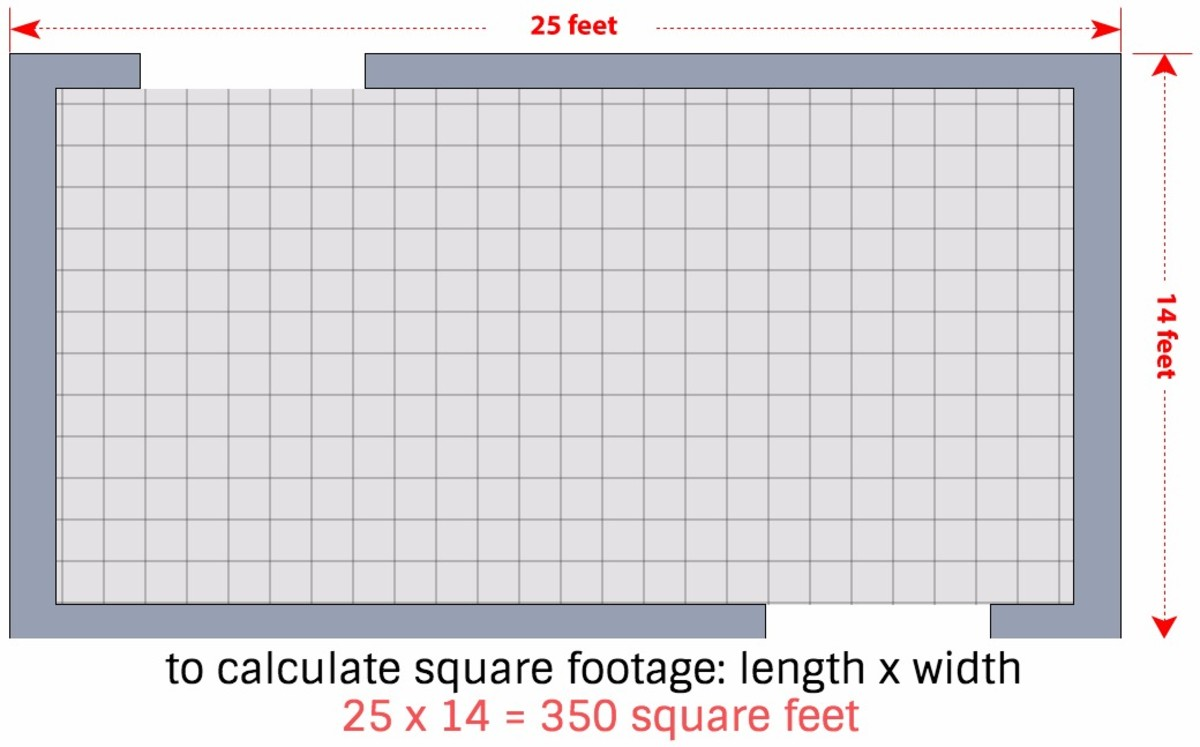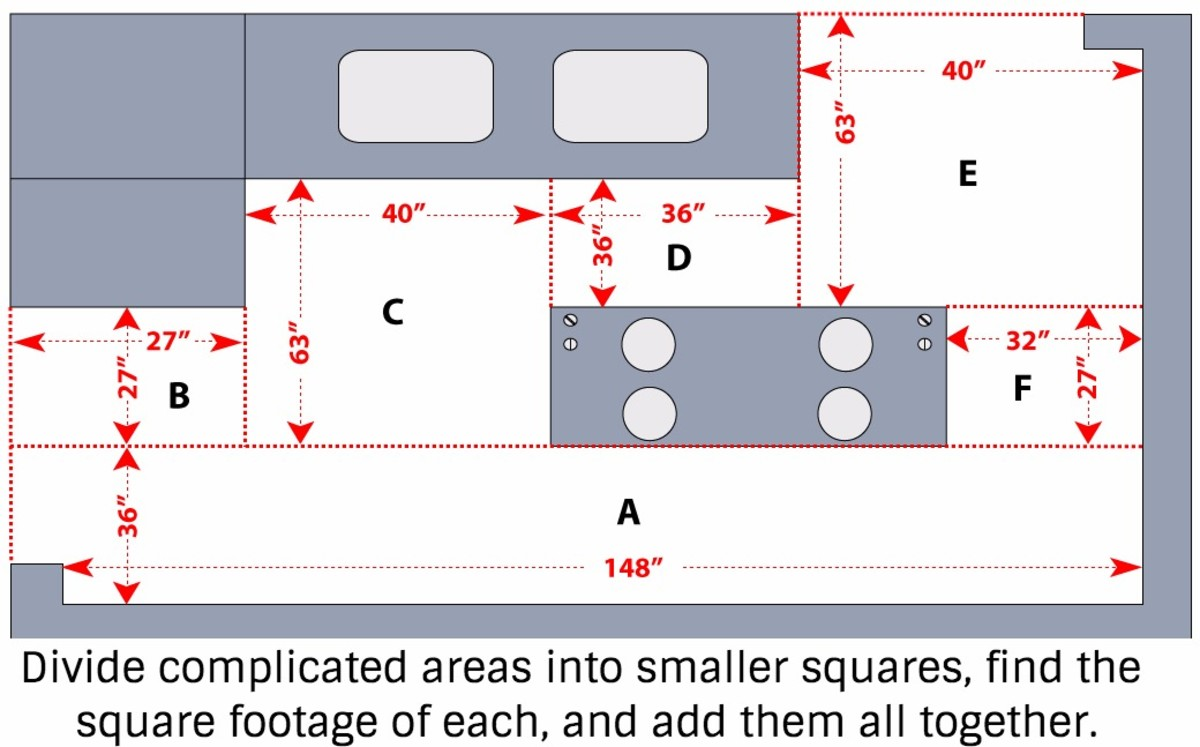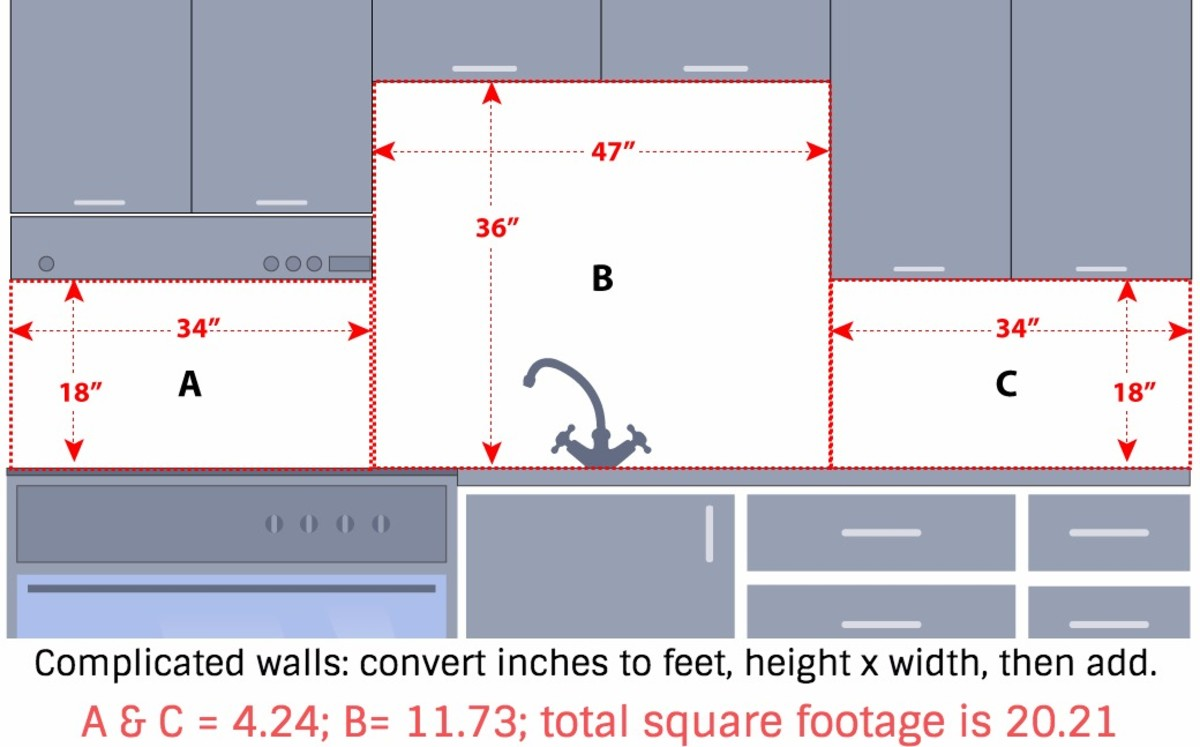# 5 Steps to Calculate How Much Tile You Need

I have over 30 years of experience providing quality floor installation and remodeling kitchens and bathrooms.How much tile do I need? Here's an easy calculation to find out.Pixabay

## How Much Tile Do I Need?

Whether you’re planning on installing your own tile floor or simply calculating the materials you need to budget for, you can determine how much tile you need. If it is a wall project instead of a floor, the steps are the same.

With a measuring tape and some simple math, you can estimate how much tile you need and how much the project is going to cost you.

What You'll Need:

• Paper
• Pen or pencil
• Measuring tape
• Calculator (optional)If you're measuring in feet, it's this easy. If you're measuring in inches, you'll have to divide each measurement by 12 before multiplying to get the square footage.

## How to Calculate How Much Tile You'll Need

1. Measure the length of one side of the room. If it is a wall project, then measure the length of the wall running up and down.
2. Measure the length of the other side of the room. If it is wall project, then measure the length of the wall running from left to right.
3. Multiply the two measurements. Multiplying the length by the width provides you with the square footage of the project area. For example, if the room measures 10 feet wide by 12 feet long, multiply 10 by 12. The square footage of this room is 120 square feet.
4. Translate the square footage to the amount of tile you need. Tile typically comes in boxes, and you have to buy the whole box. Divide the total square footage of the room by the total square footage of the tile in the box. In our example, let's say each box contains 10 square feet of tile. So if you need 120 square feet, 10 goes into 120 12 times, so you'll need to buy at least 12 boxes.
5. Calculate the overage you'll need. You should never buy just the amount of tile you need since you also need a bit extra for cuts, waste, breaks, and mistakes. Multiply the square footage of the room by 10%, then add this amount to the total square of the room. This is the total amount of square footage you should buy. To continue with the example we started above, .10 x 120 = 12, and 120 + 12 = 132. We'll need 132 square feet of tile.

Another reason to buy extra is if the style or color is discontinued and you need to replace a broken tile or two, you will need extras on hand to make the repairs. If you don't, then in order to fix it, you will have to replace the entire floor.

The moral of the story is: Spend a little bit more up front to save money in the long run.To find the square footage of area A, first convert inches to feet: 146 divided by 12 = 12.33 and 36 divided by 12 = 3, so the square footage of A is 36.99 (width x height in feet).

## What if the wall isn't a simple rectangle? What if the room isn't square?

If the space you want to tile is an unusual shape, draw a picture of the floor plan on a piece of paper, then separate the room into squares or rectangles (like I did in the diagrams on this page). Then measure each squared-off section separately, convert inches to feet (divide by 12; you'll probably end up with a decimal), multiply the length and width of each space, then add all these numbers together to find the total square footage.

## What if I need to measure in inches? What if I want an estimate that's more exact?

You'll need to convert inches to feet in order to figure out how much tile you'll need. Let's say your room is 15 feet and 8 inches long (188 inches; divide by 12 to get 15.66 feet) and 10 and a half feet wide (126 inches or 10.5 feet), then you multiply these two decimals and get 164.45 feet. You'll need 165 square feet of tile.

## How many square feet of tile comes in a box?

Most boxes hold 10 square feet, but check your box to make sure.

## How many boxes of tile will I need?

Divide the total square footage you need by the amount included in the box of tile. If you need 165 square feet, and each box holds 10, then divide 10 into 165: You'll need 16.5 boxes. So buy 17! Or 18, just to be sure.

Scroll to Continue

## Does the size or shape of the tile matter in my calculation?

No, the just the square footage matters.For the sq.ft. of areas A & C, divide 34 by 12 (4.24), divide 18 by 12 (1.5), then multiply: A & C are 6.36 sq.ft. For area B: divide 47 by 12 (3.91) and 36 by 12 (3), then multiply. B = 11.73. Add all three: 4.24+11.73+4.24 = 20.21 total sq. feet.

## Pro Tips

• A good rule of thumb is to buy an extra box of tile, just in case. Keep them in storage in case a tile breaks and needs to be replaced.
• This calculation can be applied to tile in any shape or pattern—straight, diagonal, diamond, etc. The only difference is in calculating the extra tile. Add 15% to the total square footage for a fancy shape or pattern.

This article is accurate and true to the best of the author’s knowledge. Content is for informational or entertainment purposes only and does not substitute for personal counsel or professional advice in business, financial, legal, or technical matters.

HILLARY JUMA on May 26, 2020:

awasome

Bosco on April 20, 2020:

Laying square tiles diagonally is not 45 degrees! It is 90 degrees.

King Isaac on April 20, 2020:

Add it been I have 135squaremetre how can I know the number of boxes to buy?

MD akash shammed babu on March 14, 2020:

My bathroom size 4*5 Hight 7 ft. How to measure for wall tails.

ja on February 14, 2020:

Ther is a spelling error in the last post.

aj on August 14, 2019:

Ther is a mistake in last step 120 + 12 is 132 not 122. So we need 132 square feet of tile.

VJ on July 30, 2019:

I've 96 boxes of ceramic floor tile. There is 5 pieces of 16 x 16 tile to a boxes. How much floor will the tile coverage in square feet

Smokey on March 28, 2019:

I have 1700 sf and customer bought 1700 sf but there was 14 boxes left over ( wood planks. Randome set) i use cut pieces to start another row. So does that box contain sf

kuami chosen on March 17, 2019:

nice,but can i get video

Katherine on March 06, 2019:

I have 405 feet in my house we're I will be putting tile . How many boxes of 18 in tiles will I need ? Can you please help .

sydney Hankalu on December 26, 2018:

it's my first time putting Wall tiles on my own. I need help on how to calculate the amount of tiles I need

Touching 80 going doolally on September 17, 2018:

How many wall tiles will I need for a room 8' high x 15' long x 4' wide

Carlos on September 11, 2018:

@Derrick odei: Base on high school, for example let's say your bathroom main wall is 5 feet high by 8 feet wide. Multiply hight by width to get surface area. 5 x 8= 40 feet. Now determine which tile you are going to need in order to figure out what you need in tiles. For example: 4 inch tile is equal to 1/3 as a fraction, 6 inch tile is 1/2 as a fraction and 9 inch tile is 3/4 as a fraction. So, let's say you want to use 4 inch tile. Grab your calculator and divide your surface area by 1/3 square inch like this 5 x 8 = 40 press division symbol then enter # 1 on your calculator then abc then 4 then square root symbol and then press on the equal sign = to get final result. Make sure to have a scientific calculator such as Casio f-260 solar.

Carl on August 21, 2018:

Using 12 “ tiles with 6 “ tiles how do you figure how many of each will be needed?

Derrick odei on July 14, 2018:

how to estimate wall tile

Clement on March 21, 2018:

Please I need more experience for new people so that I can learn more about how to calculate dance floor tiles and wall tiles please help me

Michael Roberts on February 07, 2018:

Hahaha 120+12+122???? Is this a different form of math, we haven't seen, or some sort of tile math? Do we add 10% then deduct 90% of that 10% then add the result to the tile totals?

Matt w on December 12, 2017:

Lol check the math in this blog you said 120 + 12 = 122???.....

Brian on October 26, 2017:

What percentage increase in estimating the amount of tile needed if the pattern is diagonal?

prettyajax on October 25, 2017:

if my room measures 5metres width by 3metres long how many boxes of tiles will l

need

David on August 29, 2017:

3300×3150

Donna on August 23, 2017:

If my room measures 15,wide by 35 feet long how many boxes of tile will I need

Blackmajesty1804@Gmail. Com on August 18, 2017:

How many cases tile I need for a 12×16 room

Sheh on August 03, 2015:

Very good info. Thanks. I go with 20% more.